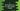# C program to find the hypotenuse of a right-angled triangle## Find the hypotenuse of a right-angled triangle:

hypot is a method defined in math.h header file in C and using this method, we can find the hypotenuse length of a right-angled triangle.

Hypotenuse is the longest side of a right-angled triangle. It is the square root of the sum of squares of other two sides.

For example, if a and b are the other two sides, hypotenuse will be square root of x^2+y^2.

hypot method takes two parameters, i.e. the adjacent and opposite side lengths. It returns the length of hypotenuse.

In this post, we will learn how to use hypot with examples.

## Definition of hypot:

hypot is defined as like below:

``````float hypotf(float a, float b);
double hypot(double a, double b);
long double hypotl(long double a, long double b);``````

Here, a and b are the two sides of the triangle. It returns the size of the hypotenuse.

## Example of hypot:

Let’s take a look at the below example:

``````#include <stdio.h>
#include <math.h>

int main()
{
double aDouble = 4;
double bDouble = 4;

double doubleHypot = hypot(aDouble, bDouble);

float aFloat = 4;
float bFloat = 4;

float floatHypot = hypot(aFloat, bFloat);

printf("%.2lf\n",doubleHypot);
printf("%.2f\n",floatHypot);

return 0;
}``````

Here, I am showing how to use hypot with double and float values.

### How to use with integer:

We can use this with integer, but you will not get accurate result. For example:

``````#include <stdio.h>
#include <math.h>

int main()
{
int aInt = 4;
int bInt = 4;

int intHypot = hypot(aInt, bInt);

printf("%d\n",intHypot);

return 0;
}``````

It will return 5.